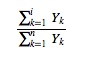# Contrast: Definition

Share on

In statistics, a contrast is a linear combination of variables such that all the coefficients add up to zero. One way to think of it is as a set of weighted variables.

In statistical tests, they define specific comparisons between variables. This might be scores, means or something else. You choose the weighting based on what you want to compare and analyze.

## A Simple Contrast

Suppose you have four samples, with means {Y1, Y2, Y3, Y4}. These are your four variables. To compare them, you design a linear combination of them with values that emphasize what you want to be looking at. If a comparison between the first and second sample means is what you are interested in, we could assign the coefficients 1, -1, 0, and 0 (since 1 – 1 + 0 + 0 = 0 is 0 adds up to zero). Then the equation of our contrast would be

(1)Y1 + (-1)Y2 + (0)Y3 + (0)Y4

or, more simply

Y1 -Y2.

Another example is 0, 2, -1, -1; this would go with the equation (0)Y1 + (2)Y2 + (-1)Y3 + (-1)Y4. Here again everything adds up to 0: 2 – 1 – 1 + 0 = 0. While it is a perfectly valid contrast, it’s not any use for comparing Y1 and Y2. Therefore, you have to choose your parameters with care.

## Important Types of Contrast

Two contrasts are orthogonal if the cross-products of the coefficients add up to zero. We can write this asIf every distinct pair in a set of contrasts is orthogonal, the set is a set of orthogonal contrasts.

Orthogonal contrasts such that the sum of the squares of the coefficients in each individual contrast add up to 1 are called orthonormal contrasts.

If an orthogonal contrast tests polynomial patterns for a set of data that has at least three means, we call it a polynomial contrast.

## References

1. Abdi & Williams. Contrast Analysis. In Neil Salkind (Ed.), Encyclopedia of Research Design. Thousand Oaks, CA: Sage. 2010. Retrieved from https://pdfs.semanticscholar.org/c0ba/1c28b0e120a459820bfb20d430fa442ebd96.pdf on July 15, 2018.
2. Gonzalez, Richard. Lecture Notes on Contrasts and Post Hoc Tests. Retrieved from http://www-personal.umich.edu/~gonzo/coursenotes/file3.pdf on July 15, 2018
CITE THIS AS:
Stephanie Glen. "Contrast: Definition" From StatisticsHowTo.com: Elementary Statistics for the rest of us! https://www.statisticshowto.com/contrast-definition/
---------------------------------------------------------------------------Need help with a homework or test question? With Chegg Study, you can get step-by-step solutions to your questions from an expert in the field. Your first 30 minutes with a Chegg tutor is free!